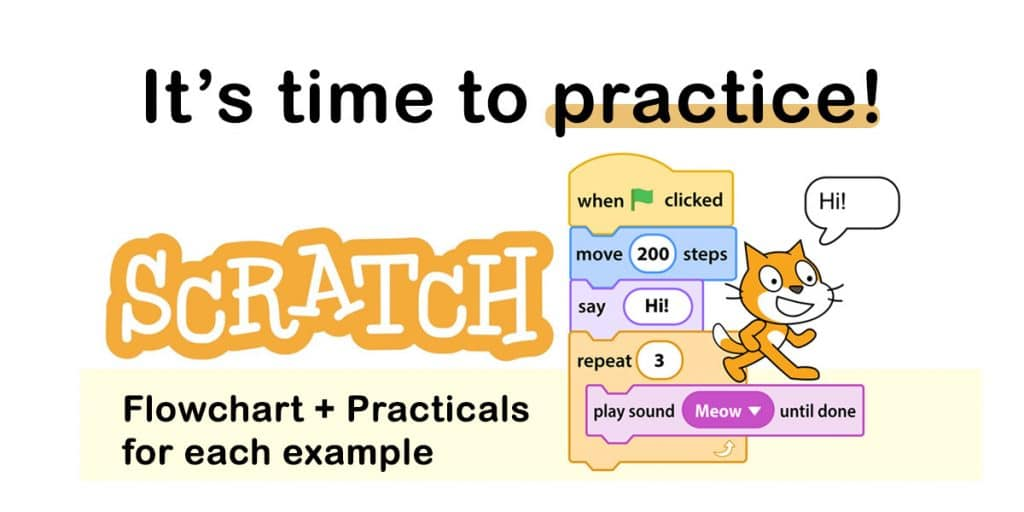Scratch is a visual programming language for students aged 8 – 16 to learn programming concepts and improve their problem-solving ability. The Scratch was developed in MIT Media Lab, and currently, it is translated into 70 different languages. Children from every corner of the world enjoy doing Scratch practicals. Now it’s time for us to enjoy coding with Scratch. Here we create flowcharts and then develop Scratch code for each flowchart. We can identify three control structures in programming. Let’s do examples for each control structure type. (Please note all the codes developed using Scratch 3)

You can use the online Scratch editor for the practicals.

Scratch Programming and Flowcharts Quiz (English Medium)

Scratch Programming and Flowcharts Quiz (Sinhala Medium)

## Scratch Practicals for Sequential Flowcharts

To print your name first you need to input your name and then display it in the output.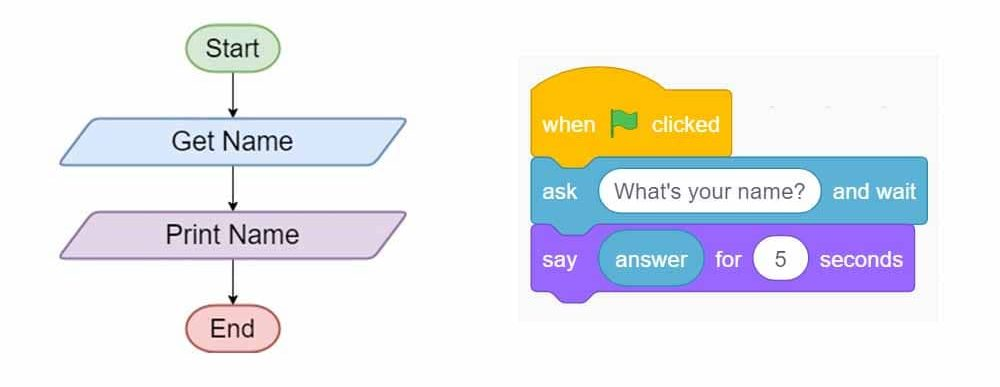Display multiplication of two numbers

Here in this code we try to get two numbers as inputs and then display the multiplied value as the answer.Find the sum of two numbers

First you need to input two numbers and then compute the summation using “Operator” code blocks in block area.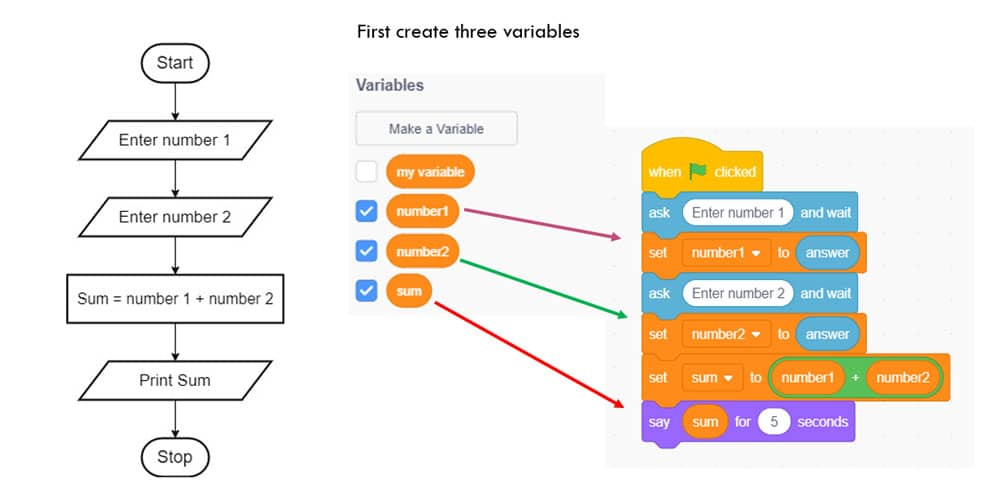Find the perimeter of a rectangle

When we input length and width of a rectangle this code displays the Perimeter of rectangle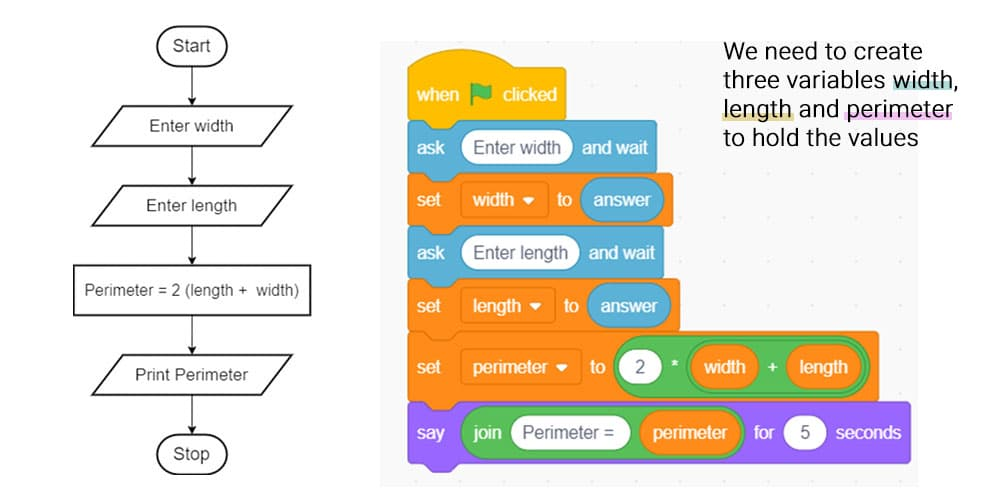Find the average of three subjects in monthly test.

Assume you need to find the average of Mathematics, English and ICT in your monthly test marks. Here you can input marks for each subject and then find the average.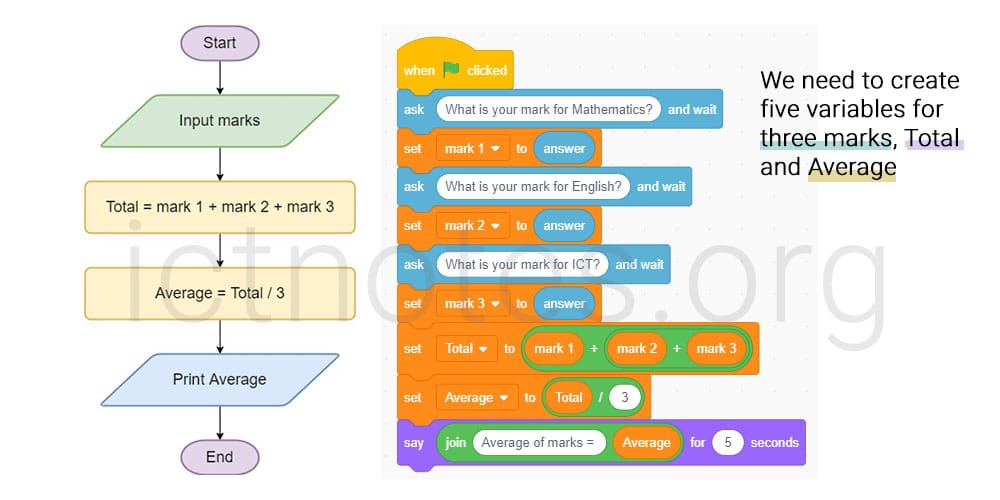To build the above programs, you will need a basic understanding of Scratch coding and flowcharts. These Scratch practicals are mainly designed for students to learn flowcharts and Scratch together.